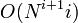# Black-box group algorithm for minimum size generating set-finding problem

## Summary

Item Value
Problem being solved generating set-finding problem: given an encoding of a group of order$N$, find a generating set for the group of size at most$\log_2 N$.
Actually, this algorithm does better: it finds a generating set of minimum size. [SHOW MORE]
Input format An encoding of a group$G$ where$G$ is a finite group (i.e.,$G$ is to be treated as a black-box group).
Output format A subset of$G$, given as a list of encodings of elements.
Guaranteed (general) properties of output generating set Size equal to the minimum size of generating set for$G$.
Size at most$\log_2 N$ where$N$ is the order of$G$.
Mode of input processing Makes queries to the black-box group operations throughout the algorithm.
Competing algorithms black-box group algorithm for small generating set-finding problem: This outputs a generating set of size at most$\log_2N$, but this need not be a generating set of minimum size.
Running time If the order of the group is$N$, and the minimum size of generating set is$s$, then ($O(N^{s+1}s)$ times the time for group operations) + (time taken to enumerate all group elements). In the worst case,$N^{s+1}s = N^{\log_2 N + 1}\log_2N$.

## Faster algorithms for some groups

The black-box group algorithm for small generating set-finding problem has a minimal generating set version that always outputs a minimal generating set, i.e., a generating set in which no element is redundant. The algorithm is faster: it takes time$O(N\operatorname{polylog}N)$ plus the time taken for enumerating all elements. For an arbitrary finite group, a minimal generating set need not have minimum size. However, if a finite group is also a group with fixed size of minimal generating set, then all minimal generating sets have the same size, which is equal to the minimum size of generating set. Thus, for such groups, the alternate algorithm is faster and still yields a generating set of minimum size.

In particular, by Burnside's basis theorem, any group of prime power order is a group with fixed size of minimal generating set, so the alternate algorithm is always preferable for such a group.

## Idea and outline

The idea is to use a deterministic many-one reduction to the generating set-checking problem, and then use the black-box group algorithm for finding the subgroup generated by a subset.

The outer loop of the algorithm traverses over$i = 0,1,2,\dots$. For each choice of$i$, the middle loop traverses over all subsets of$G$ of size$i$. There are$O(N^i)$ such subsets. The innermost loop of the algorithm runs the black-box group algorithm for finding the subgroup generated by the currently chosen subset, and checks at the end whether that subgroup is the whole group. The time taken by the innermost loop is$O(Ni)$, so the overall time taken by the intermediate loop is$O(N^{i+1}i)$. We keep doing this until we hit upon a subset that generates the whole group. This inevitably happens for$i \le \log_2N$.

In order terms, the sum of the times taken for all smaller sized subsets is of a smaller order of magnitude than the time taken for subsets of a given size, so the critical determinant of running time is the time taken by subsets of size$s$ where$s$ is the minimum size of generating set.

It is possible to make some improvements in the actual implementation of the algorithm but these minor improvement do not yield improvements in the order of magnitude of the running time. Some possible improvements include remembering which sets of a given size are irredundant generating sets for the subgroup they do generate, and trying to add new elements only to those subsets.